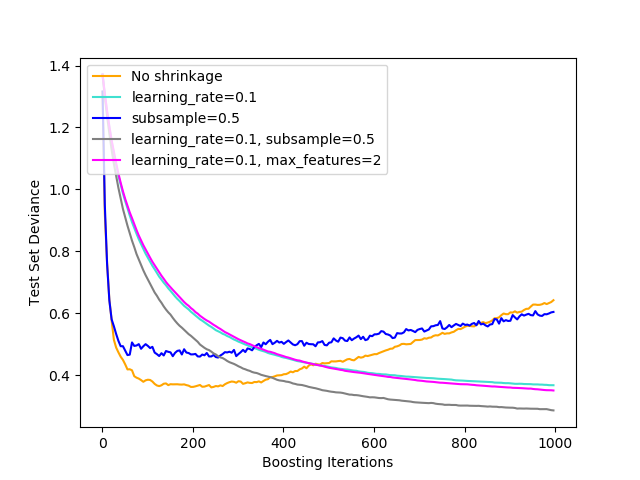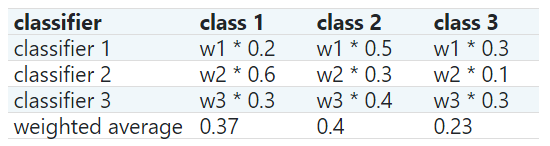1.11 集成算法¶

• 平均法（averaging methods)中，该方法的原理是构建多个独立的估计器，然后取它们的预测结果的平均。一般来说，组合之后的估计器是会比单个估计器要好的，因为它的方差减小了。

• 相反，在提升法(boosting methods)中，基估计器是按顺序建立的，并且试图减小组合估计器的偏差。其动机是将几个弱模型结合起来，形成一个强大的整体。

1.11.1 Bagging meta-estimator(Bagging 元-估计器)

Bagging方法有许多不同的变种(flavours)，但主要是因为它们提取训练集的随机子集的方式不同：

• 如果抽取的数据集的随机子集是样本的随机子集，我们叫做粘贴 (Pasting) [B1999] 。
• 如果样本抽取是有放回的，我们称为 Bagging。 [B1996]
• 如果抽取的数据集的随机子集是特征的随机子集，我们叫做随机子空间 (Random Subspaces) [H1998]
• 最后，如果基估计器构建在对于样本和特征抽取的子集之上时，我们叫做随机补丁 (Random Patches) [LG2012].

>>> from sklearn.ensemble import BaggingClassifier
>>> from sklearn.neighbors import KNeighborsClassifier
>>> bagging = BaggingClassifier(KNeighborsClassifier(),
...                             max_samples=0.5, max_features=0.5)

[B1999] L. Breiman, “Pasting small votes for classification in large databases and on-line”, Machine Learning, 36(1), 85-103, 1999.

[B1996] L. Breiman, “Bagging predictors”, Machine Learning, 24(2), 123-140, 1996.

[H1998] T. Ho, “The random subspace method for constructing decision forests”, Pattern Analysis and Machine Intelligence, 20(8), 832-844, 1998.

[LG2012] G. Louppe and P. Geurts, “Ensembles on Random Patches”, Machine Learning and Knowledge Discovery in Databases, 346-361, 2012.

1.11.2 由随机树组成的森林

sklearn.ensemble集成模块包括两种基于随机决策树的平均算法：RandomForest算法和Extra-Trees算法。这两种算法都是专门为树设计的扰动和组合技术(perturb-and-combine techniques)[B1998] 。这意味着在分类器构造过程中引入随机性来创建一组不同的分类器的集合。集成之后的预测是每个分类器的平均。

>>> from sklearn.ensemble import RandomForestClassifier
>>> X = [, ]
>>> Y = 
>>> clf = RandomForestClassifier(n_estimators=10)
>>> clf = clf.fit(X, Y)

1.11.2.2 极端随机树

>>> from sklearn.model_selection import cross_val_score
>>> from sklearn.datasets import make_blobs
>>> from sklearn.ensemble import RandomForestClassifier
>>> from sklearn.ensemble import ExtraTreesClassifier
>>> from sklearn.tree import DecisionTreeClassifier

>>> X, y = make_blobs(n_samples=10000, n_features=10, centers=100,
...     random_state=0)

>>> clf = DecisionTreeClassifier(max_depth=None, min_samples_split=2,
...     random_state=0)
>>> scores = cross_val_score(clf, X, y, cv=5)
>>> scores.mean()
0.98...

>>> clf = RandomForestClassifier(n_estimators=10, max_depth=None,
...     min_samples_split=2, random_state=0)
>>> scores = cross_val_score(clf, X, y, cv=5)
>>> scores.mean()
0.999...

>>> clf = ExtraTreesClassifier(n_estimators=10, max_depth=None,
...     min_samples_split=2, random_state=0)
>>> scores = cross_val_score(clf, X, y, cv=5)
>>> scores.mean() > 0.999
True

1.11.2.4 并行化

[B2001] Breiman, “Random Forests”, Machine Learning, 45(1), 5-32, 2001.

[B1998] Breiman, “Arcing Classifiers”, Annals of Statistics 1998.

P. Geurts, D. Ernst., and L. Wehenkel, “Extremely randomized trees”, Machine Learning, 63(1), 3-42, 2006.

1.11.2.5 特征重要性评估

[L2014] G. Louppe, “Understanding Random Forests: From Theory to Practice”, PhD Thesis, U. of Liege, 2014.

1.11.2.6 完全随机树嵌入

RandomTreesEmbedding 实现了一个无监督的数据转换。 通过由完全随机树构成的森林，RandomTreesEmbedding 使用数据最终归属的叶子节点的索引值对数据进行编码。 该索引以 one-of-K 方式编码，最终形成一个高维的稀疏二值化编码。 这种编码可以被非常高效地计算出来，并且可以作为其他学习任务的基础。 编码的大小和稀疏度可以通过选择树的数量和每棵树的最大深度来确定。对于集成中的每棵树的，每个样本对应其中的一个叶节点。 编码的大小（维度）最多为 n_estimators * 2 ** max_depth ，即森林中的叶子节点的最大数。

sklearn.ensemble 模块包含了流行的提升算法 AdaBoost, 这个算法是由 Freund 和 Schapire 在 1995 年提出来的[FS1995]。

AdaBoost 的核心思想是用反复调整的数据来训练一系列的弱学习器(一个弱学习器模型仅仅比随机猜测好一点, 比如一个简单的决策树),由这些弱学习器的预测结果通过加权投票(或加权求和)的方式组合, 产生最终的预测结果。在每一次所谓的提升（boosting）迭代中，数据的修改由应用于每一个训练样本的（新） 的权重 组成。 初始化时,将所有弱学习器的权重都设置为 ,因此第一次迭代仅仅是通过原始数据训练出一个弱学习器。在接下来的连续迭代中,样本的权重逐个地被修改,学习算法也因此要重新应用这些已经修改的权重的数据。在给定的一个迭代中, 那些在上一轮迭代中被预测为错误结果的样本的权重将会被增加，而那些被预测为正确结果的样本的权重将会被降低。随着迭代次数的增加，那些难以预测的样例的影响将会越来越大，每一个随后的弱学习器都将会被强迫更加关注那些在之前被错误预测的样例[HTF]。AdaBoost可以用于分类和回归问题：

1.11.3.1 使用方法

>>> from sklearn.model_selection import cross_val_score

>>> scores = cross_val_score(clf, X, y, cv=5)
>>> scores.mean()
0.9...

• [FS1995] Y. Freund, and R. Schapire, “A Decision-Theoretic Generalization of On-Line Learning and an Application to Boosting”, 1997.
• [ZZRH2009] J. Zhu, H. Zou, S. Rosset, T. Hastie. “Multi-class AdaBoost”, 2009.
• [D1997] Drucker. “Improving Regressors using Boosting Techniques”, 1997.
• HTF(1,2,3) T. Hastie, R. Tibshirani and J. Friedman, “Elements of Statistical Learning Ed. 2”, Springer, 2009.

1.11.4 梯度树提升

1.11.4.1 分类

>>> from sklearn.datasets import make_hastie_10_2

>>> X, y = make_hastie_10_2(random_state=0)
>>> X_train, X_test = X[:2000], X[2000:]
>>> y_train, y_test = y[:2000], y[2000:]

...     max_depth=1, random_state=0).fit(X_train, y_train)
>>> clf.score(X_test, y_test)
0.913...

1.11.4.2 回归

>>> import numpy as np
>>> from sklearn.metrics import mean_squared_error
>>> from sklearn.datasets import make_friedman1

>>> X, y = make_friedman1(n_samples=1200, random_state=0, noise=1.0)
>>> X_train, X_test = X[:200], X[200:]
>>> y_train, y_test = y[:200], y[200:]
...     max_depth=1, random_state=0, loss='ls').fit(X_train, y_train)
>>> mean_squared_error(y_test, est.predict(X_test))
5.00...

1.11.4.3 训练额外的弱学习器

>>> _ = est.set_params(n_estimators=200, warm_start=True)
# set warm_start and new nr of trees
>>> _ = est.fit(X_train, y_train) # fit additional 100 trees to est
>>> mean_squared_error(y_test, est.predict(X_test))
3.84...

1.11.4.5 数学公式

1.11.4.5.1 回归

GBRT回归器是一种加法模型，其对给定输入的预测由以下形式给出：

1.11.4.6 损失函数

• 回归
• 最小二乘('ls')：回归的自然选择，因为它具有优越的计算特性。用目标值的平均值给出了初始模型。
• Huber(‘Huber’)：另一个结合最小二乘和最小绝对偏差的稳健损失函数；使用alpha控制异常值的灵敏度(详见[F2001])。
• Quantile ('quantile'):分位数回归的损失函数。使用 0 < alpha < 1指定分位数。此损失函数可用于创建预测间隔 (参见梯度提升回归的预测间隔 Prediction Intervals for Gradient Boosting Regression)
• 分类
• 二项偏差('deviance')：二分类的负二项式对数似然损失函数(提供概率估计)。用对数比率给出了初始模型。
• 多项偏差('deviance')：对于多分类问题的负的多项对数似然损失函数具有 n_classes个互斥的类。提供概率估计。 初始模型由每个类的先验概率给出.在每一次迭代中 n_classes 回归树被构建,这使得 GBRT 在处理多类别数据集时相当低效。
• 指数损失('exponential')：与 AdaBoostClassifier 具有相同的损失函数。与 'deviance' 相比，对被错误标记的样本的鲁棒性较差，仅用于在二分类问题。

1.11.4.7 学习收缩率

[F2001] 提出了一种简单的正则化策略，通过常数因子 来衡量每个弱学习器的贡献：

1.11.4.8 子采样(Subsampling)

[F1999] 提出了随机梯度提升,这种方法将梯度提升（gradient boosting）和 bootstrap averaging(bagging) 相结合。在每次迭代中,基分类器是通过抽取所有可利用训练集中一小部分的 subsample 训练得到的子样本采用无放回的方式采样。 subsample 参数的值一般设置为 0.5 。另一种降低方差的策略是对随机森林分类器中类似随机分裂的特征进行二次采样。通过max_features参数可以控制子采样特征的数量。

1.11.4.9 特征重要性的解释

>>> from sklearn.datasets import make_hastie_10_2

>>> X, y = make_hastie_10_2(random_state=0)
...     max_depth=1, random_state=0).fit(X, y)
>>> clf.feature_importances_
array([0.10..., 0.10..., 0.11..., ...

1.11.5 基于直方图的梯度提升

>>> # explicitly require this experimental feature
>>> from sklearn.experimental import enable_hist_gradient_boosting  # noqa
>>> # now you can import normally from ensemble

1.11.5.1 使用方法

>>> from sklearn.datasets import make_hastie_10_2

>>> X, y = make_hastie_10_2(random_state=0)
>>> X_train, X_test = X[:2000], X[2000:]
>>> y_train, y_test = y[:2000], y[2000:]

>>> clf.score(X_test, y_test)
0.8965

l2_regularization参数是损失函数上的正则化参数，对应于[XGBoost]方程(2)中的

1.11.5.2 缺失值支持

>>> from sklearn.experimental import enable_hist_gradient_boosting  # noqa
>>> import numpy as np

>>> X = np.array([012, np.nan]).reshape(-11)
>>> y = 

>>> gbdt.predict(X)
array()

>>> X = np.array([0, np.nan, 12, np.nan]).reshape(-11)
>>> y = 
...                                       max_depth=2,
...                                       learning_rate=1,
...                                       max_iter=1).fit(X, y)
>>> gbdt.predict(X)
array()

1.11.5.3 样本加权支持

>>> X = [,
...      ,
...      ,
...      ]
>>> y = 
>>> # ignore the first 2 training samples by setting their weight to 0
>>> sample_weight = 
>>> gb.fit(X, y, sample_weight=sample_weight)
>>> gb.predict([])
array()
>>> gb.predict_proba([])
0.99...

1.11.5.4 单调约束

, 其中是具有两个特征的预测器。

>>> from sklearn.experimental import enable_hist_gradient_boosting  # noqa

... # positive, negative, and no constraint on the 3 features

1.11.5.5 低级并行

• 将样本从真实值映射到整数值箱(然而，找到bin阈值是连续的)
• 构建直方图在特征上并行化
• 在节点上找到最佳分割点的方法是在特征上并行化
• 在fit期间，将样本映射到左、右两个子节点，并在样本上并行化
• 梯度和Hessians计算在样本上并行化
• 预测在样本上并行化

1.11.5.6 为什么它更快

[F1999] Friedmann, Jerome H., 2007, “Stochastic Gradient Boosting”

[R2007] G. Ridgeway, “Generalized Boosted Models: A guide to the gbm package”, 2007

[XGBoost] Tianqi Chen, Carlos Guestrin, “XGBoost: A Scalable Tree Boosting System”

LightGBM(1,2) Ke et. al. “LightGBM: A Highly Efficient Gradient BoostingDecision Tree”

1.11.6 投票分类器

VotingClassifier （投票分类器）的原理是结合了多个不同的机器学习分类器,并且采用多数表决（majority vote）（硬投票） 或者平均预测概率（软投票）的方式来预测分类标签。 这样的分类器可以用于一组同样表现良好的模型,以便平衡它们各自的弱点。

1.11.6.1 多数类标签(多数/硬投票)

• classifier 1 -> class 1
• classifier 2 -> class 1
• classifier 3 -> class 2

VotingClassifier( voting='hard')将根据多数类标签将示例分类为 “class 1”。

• classifier 1 -> class 2
• classifier 2 -> class 1

1.11.6.2 使用方法

>>> from sklearn import datasets
>>> from sklearn.model_selection import cross_val_score
>>> from sklearn.linear_model import LogisticRegression
>>> from sklearn.naive_bayes import GaussianNB
>>> from sklearn.ensemble import RandomForestClassifier
>>> from sklearn.ensemble import VotingClassifier

>>> X, y = iris.data[:, 1:3], iris.target

>>> clf1 = LogisticRegression(random_state=1)
>>> clf2 = RandomForestClassifier(n_estimators=50, random_state=1)
>>> clf3 = GaussianNB()

>>> eclf = VotingClassifier(
...     estimators=[('lr', clf1), ('rf', clf2), ('gnb', clf3)],
...     voting='hard')

>>> for clf, label in zip([clf1, clf2, clf3, eclf], ['Logistic Regression''Random Forest''naive Bayes''Ensemble']):
...     scores = cross_val_score(clf, X, y, scoring='accuracy', cv=5)
...     print("Accuracy: %0.2f (+/- %0.2f) [%s]" % (scores.mean(), scores.std(), label))
Accuracy: 0.95 (+/- 0.04) [Logistic Regression]
Accuracy: 0.94 (+/- 0.04) [Random Forest]
Accuracy: 0.91 (+/- 0.04) [naive Bayes]
Accuracy: 0.95 (+/- 0.04) [Ensemble]

1.11.6.3 加权平均概率(软投票)这里，预测的类标签是2，因为它具有最高的平均概率。

>>> from sklearn import datasets
>>> from sklearn.tree import DecisionTreeClassifier
>>> from sklearn.neighbors import KNeighborsClassifier
>>> from sklearn.svm import SVC
>>> from itertools import product
>>> from sklearn.ensemble import VotingClassifier

>>> X = iris.data[:, ]
>>> y = iris.target

>>> # Training classifiers
>>> clf1 = DecisionTreeClassifier(max_depth=4)
>>> clf2 = KNeighborsClassifier(n_neighbors=7)
>>> clf3 = SVC(kernel='rbf', probability=True)
>>> eclf = VotingClassifier(estimators=[('dt', clf1), ('knn', clf2), ('svc', clf3)],
...                         voting='soft', weights=)

>>> clf1 = clf1.fit(X, y)
>>> clf2 = clf2.fit(X, y)
>>> clf3 = clf3.fit(X, y)
>>> eclf = eclf.fit(X, y)

1.11.6.4 投票分类器（VotingClassifier）在网格搜索（GridSearchCV）的应用

VotingClassifier还可以与 GridSearchCV一起使用，以调整单个估计器的超参数：

>>> from sklearn.model_selection import GridSearchCV
>>> clf1 = LogisticRegression(random_state=1)
>>> clf2 = RandomForestClassifier(random_state=1)
>>> clf3 = GaussianNB()
>>> eclf = VotingClassifier(
...     estimators=[('lr', clf1), ('rf', clf2), ('gnb', clf3)],
...     voting='soft'
... )

>>> params = {'lr__C': [1.0100.0], 'rf__n_estimators': }

>>> grid = GridSearchCV(estimator=eclf, param_grid=params, cv=5)
>>> grid = grid.fit(iris.data, iris.target)

1.11.6.5 使用方法

>>> eclf = VotingClassifier(
...     estimators=[('lr', clf1), ('rf', clf2), ('gnb', clf3)],
...     voting='soft'
... )

>>> eclf = VotingClassifier(
...     estimators=[('lr', clf1), ('rf', clf2), ('gnb', clf3)],
...     voting='soft', weights=[2,5,1]
... )

1.11.7 投票回归器

VotingRegressor的思想是将上不同的机器学习回归器组合起来，并返回平均预测值。这样的回归器对于一组同样表现良好的模型是有用的，以平衡它们各自的弱点。

1.11.7.1 使用方法

>>> from sklearn.ensemble import RandomForestRegressor
>>> from sklearn.linear_model import LinearRegression
>>> from sklearn.ensemble import VotingRegressor

>>> # Training classifiers
>>> reg2 = RandomForestRegressor(random_state=1, n_estimators=10)
>>> reg3 = LinearRegression()
>>> ereg = VotingRegressor(estimators=[('gb', reg1), ('rf', reg2), ('lr', reg3)])
>>> ereg = ereg.fit(X, y)

1.11.8 叠加泛化

StackingClassifier and StackingRegressor 提供了适用于分类和回归问题的策略。

estimators对应于在估计器的列表， 它们在输入数据上并行地堆叠在一起。它应该是给出名字和估计器的列表：

>>> from sklearn.linear_model import RidgeCV, LassoCV
>>> from sklearn.svm import SVR
>>> estimators = [('ridge', RidgeCV()),
...               ('lasso', LassoCV(random_state=42)),
...               ('svr', SVR(C=1, gamma=1e-6))]

final_estimator将使用 estimators的预测作为输入。当使用StackingClassifier 或者StackingRegressor, 时，它需要是一个分类器或回归器：

>>> from sklearn.ensemble import StackingRegressor
>>> reg = StackingRegressor(
...     estimators=estimators,

>>> from sklearn.model_selection import train_test_split
>>> X_train, X_test, y_train, y_test = train_test_split(X, y,
...                                                     random_state=42)
>>> reg.fit(X_train, y_train)
StackingRegressor(...)

StackingRegressor and StackingClassifier可以用作任何其他的回归者或分类器，通用方法predict, predict_proba, 和 decision_function ，例如：

>>> y_pred = reg.predict(X_test)
>>> from sklearn.metrics import r2_score
>>> print('R2 score: {:.2f}'.format(r2_score(y_test, y_pred)))
R2 score: 0.81

>>> reg.transform(X_test[:5])
array([[28.78..., 28.43...  , 22.62...],
[35.96..., 32.58..., 23.68...],
[14.97..., 14.05..., 16.45...],
[25.19..., 25.54..., 22.92...],
[18.93..., 19.26..., 17.03... ]])

>>> final_layer = StackingRegressor(
...     estimators=[('rf', RandomForestRegressor(random_state=42)),
...     final_estimator=RidgeCV()
...     )
>>> multi_layer_regressor = StackingRegressor(
...     estimators=[('ridge', RidgeCV()),
...                 ('lasso', LassoCV(random_state=42)),
...                 ('svr', SVR(C=1, gamma=1e-6, kernel='rbf'))],
...     final_estimator=final_layer
... )
>>> multi_layer_regressor.fit(X_train, y_train)
StackingRegressor(...)
>>> print('R2 score: {:.2f}'
...       .format(multi_layer_regressor.score(X_test, y_test)))
R2 score: 0.83

[W1992] Wolpert, David H. “Stacked generalization.” Neural networks 5.2 (1992): 241-259.